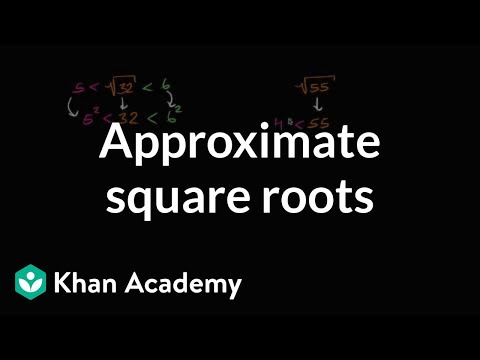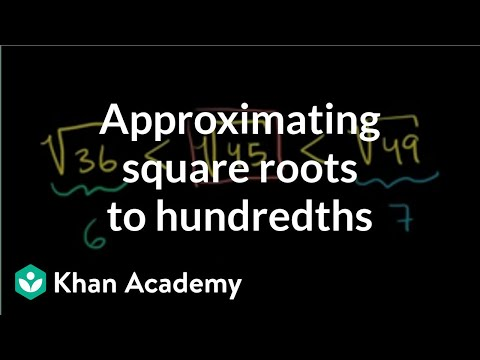Video

# Classifying numbers (Full video)

Description: Learn how to classify numbers as whole numbers, integers, rational numbers, and irrational numbers. So this circle, over here, this represents all of the numbers that can be represented as the fraction of two integers, and, of course, the denominator can't be equal to zero, because we don't know what it means to put a zero in the denominator. So, let's call these, or the standard way of calling these things. These things can be represented as a fraction of two integers, we call these rational numbers.

### Other videos you might be interested in### Approximating square roots (Full video)